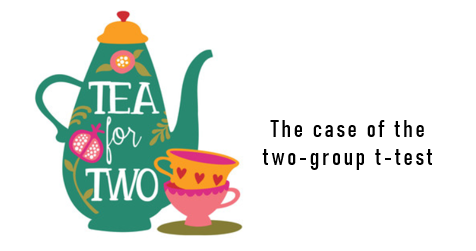# Tea for 2: The Case of the Two-Group t-TestMaria T Ping & Willy A Renandya

An independent-sample t-test is a procedure used to compare two sets of mean scores obtained from two unrelated groups of individuals. This statistical procedure is usually used in an experimental quantitative research design involving two groups: an experimental and control groups. The purpose is to determine whether the experimental group performs better than the control group.

Statisticians suggest that the t-test is applicable for data obtained from a normally distributed population. This often means before the experiment, we need to make sure that the two groups you are comparing should not be significantly different from one another and that they are selected based on the random assumption. For more information about this, please consult a standard statistics text.

Procedure

1. Prepare your two sets of scores (e.g., post-test scores) from the two groups of students in your study

2. You can calculate manually by entering the values into the independent-sample t-test formula. You can also make use of existing online programs such as VassarStats and Social Science Statistics to get a quicker and accurate result. A worked example is provided here.

3. There are a few terms you need to know with when dealing with independent-sample t-test, some of which are discussed below:

Experimental group: the group that receives a treatment e.g., innovative ways of learning vocabulary

Control group: the group that does not receive any special treatment, i.e., students normally follow regular instruction.

t computed: the t-value that you get from your calculation

t table/ critical t value: the value that we use to compare to our t computed, in order to find out if there is a statistically significant difference or not

df/ degree of freedom: for independent- sample t-test, df = total sample size (n1+n2) – 2 (because you have two groups). e.g. your experimental group (n1) is 30, your control group (n2) is 30, then your df= (30+30) -2 = 58

Level of significance: the most commonly used one is 0.05 (5%)

Probability (p) value: this value must be less than your level of significance in order to conclude that there is a significant difference

4. Interpret your results and conclude whether there is a significant difference between the two groups’ mean scores or not.  If your t computed is larger than the critical t/ t-table, the difference is statistically significant at p<.05.